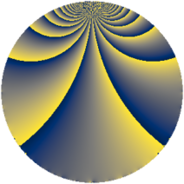# Properties

 Label 99.2.pLevel $99$ Weight $2$ Character orbit 99.p Rep. character $\chi_{99}(2,\cdot)$ Character field $\Q(\zeta_{30})$ Dimension $80$ Newform subspaces $1$ Sturm bound $24$ Trace bound $0$

# Related objects

## Defining parameters

 Level: $$N$$ $$=$$ $$99 = 3^{2} \cdot 11$$ Weight: $$k$$ $$=$$ $$2$$ Character orbit: $$[\chi]$$ $$=$$ 99.p (of order $$30$$ and degree $$8$$) Character conductor: $$\operatorname{cond}(\chi)$$ $$=$$ $$99$$ Character field: $$\Q(\zeta_{30})$$ Newform subspaces: $$1$$ Sturm bound: $$24$$ Trace bound: $$0$$

## Dimensions

The following table gives the dimensions of various subspaces of $$M_{2}(99, [\chi])$$.

Total New Old
Modular forms 112 112 0
Cusp forms 80 80 0
Eisenstein series 32 32 0

## Trace form

 $$80q - 15q^{2} - 3q^{3} + 5q^{4} - 6q^{5} - 15q^{6} - 5q^{7} - q^{9} + O(q^{10})$$ $$80q - 15q^{2} - 3q^{3} + 5q^{4} - 6q^{5} - 15q^{6} - 5q^{7} - q^{9} - 3q^{11} - 54q^{12} - 5q^{13} - 9q^{14} + 5q^{16} - 50q^{19} - 3q^{20} - 11q^{22} - 42q^{23} - 5q^{24} - 2q^{25} + 3q^{27} - 20q^{28} + 30q^{29} + 50q^{30} - 6q^{31} + 4q^{33} - 10q^{34} - 17q^{36} - 6q^{37} + 9q^{38} + 85q^{39} + 15q^{40} - 15q^{41} + 19q^{42} - 12q^{45} - 40q^{46} - 21q^{47} + 70q^{48} - q^{49} + 60q^{50} - 45q^{51} - 5q^{52} - 18q^{55} + 90q^{56} + 60q^{57} - 29q^{58} + 81q^{59} + 43q^{60} - 5q^{61} + 15q^{63} - 8q^{64} - 39q^{66} + 10q^{67} + 180q^{68} - 20q^{69} + 30q^{70} + 5q^{72} - 20q^{73} - 15q^{74} - 30q^{75} + 33q^{77} + 152q^{78} - 5q^{79} - 73q^{81} - 2q^{82} - 60q^{83} - 135q^{84} - 5q^{85} - 48q^{86} - 59q^{88} - 70q^{90} + 52q^{91} - 213q^{92} - 34q^{93} - 5q^{94} - 135q^{95} - 145q^{96} + 27q^{97} + 35q^{99} + O(q^{100})$$

## Decomposition of $$S_{2}^{\mathrm{new}}(99, [\chi])$$ into newform subspaces

Label Dim. $$A$$ Field CM Traces $q$-expansion
$$a_2$$ $$a_3$$ $$a_5$$ $$a_7$$
99.2.p.a $$80$$ $$0.791$$ None $$-15$$ $$-3$$ $$-6$$ $$-5$$